`mailto:` blog -at- heyrick -dot- eu

It's a Sunday, so I thought I'd do a 4K/2160p video again. Since I know my phone can compress a gigabyte and a quarter down to around 650MB...which only took about two and a half hours (*) to upload.

* - estimation, I was cleaning up in and around the kitchen, so I set it up, made tea, and let it get on with the upload while I got on with things.

## Mamie Fletcher's House 12

### Fun with the development builds

Prior to the Release Candidate versions of Mamie (circa version 0.20), the game was built with some extra features added to aid in testing.

### Extra key presses to do various things

Pressing the help key (F1) would show a different help screen than usual, listing the various additional keypresses available, and drawing Lucy in all her states... just because.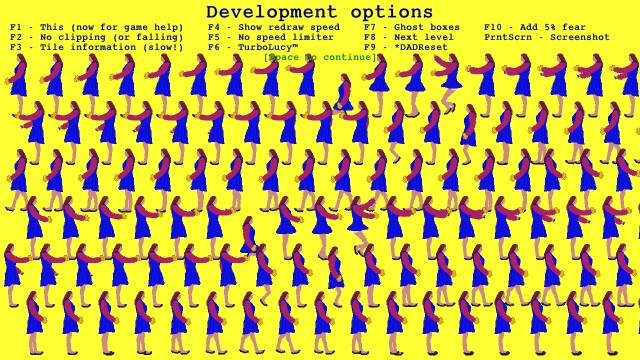The extra development options.

These extras were:

• F1 - Show help
When playing the game, F1 will show the development help. When looking at the development help, F1 will show the regular game help.
• F2 - No clipping
This means Lucy can pass through walls. It also, as a side effect, disables the falling behaviour.
• F3 - Tile information
This annotates every tile with information, as well as marking the ghosts. Because of all the extra drawing that is done, this does add a fairly significant speed hit.
• F4 - Show redraw speed
The redraw speed is always being calculated (that's how the game knows if the machine is 'too slow'). Using this option, it'll pop the speed on the upper right of the screen under the film counters. It should say on or near 50 fps when running normally.
• F5 - Toggle the speed limiter
The speed limiter tries to keep the game running at a fairly constant 50fps. Without the limiter, the only timing will be the VSync as the display is refreshed, however this can be all sorts of values, with 60 and 75 (corresponding to the monitor refresh rate in hertz) being the most common. Obviously it's just not a great idea to have the game run half again as fast as it should on a machine with a faster screen refresh, hence the speed limiter.
• F6 - TurboLucy™
This doubles Lucy's movement speed. Makes it easy to get to the far side of a level. Has some interesting side effects due to said speed.
• F7 - Ghost boxes
Toggle whether or not bounding boxes are drawn around Lucy, the ghosts, and spiders. Useful for verifying the collisions are being handled sanely.
• F8 - Next level
Set the ghost count to zero, which means the level has been completed. Because if you want to test the behaviour of level seven, you don't want to have to play the first six. Just hammer F8 and you'll be there.
When in debug mode, Mamie can spit out a lot of debug data. Therefore, sometimes it is useful to clear the DADebug log so that what's there is just what is to be examined. So I'd line up a move, say. Then I'd prod F9 to clear the log, perform the move, and then keep pressing Esc until I was back at the desktop.
• F10 - Add 5% fear
Exactly what it says on the tin.
• F11 - Set fear to zero
Oddly, I forgot to add this to the menu. Whoops. Anyway, it resets the fear to zero.
• Print Screen - Screenshot
Save the screen as a sprite, within Mamie, called "_scrHHMMSS" where the last six characters are the current time (hours, minutes, seconds).

In case you've not tried pressing random keys whilst playing Mamie, I'll let you know that F8 and PrintScreen are supported.
F8, in particular, can be useful if there's a level you are stuck on, to go practice it without having to play all the way to it. But don't bother trying to finish the game by pressing this key repeatedly. You'll get chewed out for cheating. ☺

And PrintScreen? Useful for recording your moments of epicness.

### Tile information

Lots of nice juicy detail. Useful for correlating with the debug log. Having said that, all those extra rectangles and text output (which are added on top) really slay the redraw speed.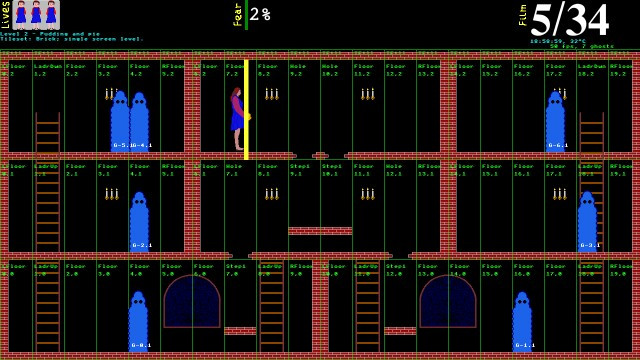Tile information.

### Debug log

Various debug options could be selectively switched on. These were for debugging movements, debugging the floor position checking, and debugging jumping/falling activity.

To give an example, here is a snippet of the debug log. All that is happening here is that I pressed the 'Up' key to make Lucy jump.

```     lucy_can_move()
floorlevel = 0, tilebase = 0
tile at 69,0 is type 12
horizontal offset in tile is 3
lucy_move_up
jumping
Trajectory left = 0, right = 0
lucy_calculate_floor()
checking: tile 69,0 is type 12
calculated floor position is 0
jump - Y = 5, motion = 65
lucy_calculate_floor()
checking: tile 69,0 is type 12
calculated floor position is 0
jump - Y = 10, motion = 60
lucy_calculate_floor()
checking: tile 69,0 is type 12
calculated floor position is 0
jump - Y = 15, motion = 55
lucy_calculate_floor()
checking: tile 69,0 is type 12
calculated floor position is 0
jump - Y = 20, motion = 50
lucy_calculate_floor()
checking: tile 69,0 is type 12
calculated floor position is 0
jump - Y = 25, motion = 45
lucy_calculate_floor()
checking: tile 69,0 is type 12
calculated floor position is 0
jump - Y = 30, motion = 40
lucy_calculate_floor()
checking: tile 69,0 is type 12
calculated floor position is 0
jump - Y = 35, motion = 35
lucy_calculate_floor()
checking: tile 69,0 is type 12
calculated floor position is 0
jump - Y = 40, motion = 30
lucy_calculate_floor()
checking: tile 69,0 is type 12
calculated floor position is 0
jump - Y = 45, motion = 25
lucy_calculate_floor()
checking: tile 69,0 is type 12
calculated floor position is 0
jump - Y = 50, motion = 20
lucy_calculate_floor()
checking: tile 69,0 is type 12
calculated floor position is 0
jump - Y = 55, motion = 15
lucy_calculate_floor()
checking: tile 69,0 is type 12
calculated floor position is 0
jump - Y = 60, motion = 10
lucy_calculate_floor()
checking: tile 69,0 is type 12
calculated floor position is 0
jump - Y = 65, motion = 5
lucy_calculate_floor()
checking: tile 69,0 is type 12
calculated floor position is 0
jump - Y = 70, motion = 0
jump: finished
jump: falling back down after jump up
lucy_calculate_floor()
checking: tile 69,0 is type 12
calculated floor position is 0
fall: Y = 65, motion = 65
lucy_calculate_floor()
checking: tile 69,0 is type 12
calculated floor position is 0
fall: Y = 60, motion = 60
lucy_calculate_floor()
checking: tile 69,0 is type 12
calculated floor position is 0
fall: Y = 55, motion = 55
lucy_calculate_floor()
checking: tile 69,0 is type 12
calculated floor position is 0
fall: Y = 50, motion = 50
lucy_calculate_floor()
checking: tile 69,0 is type 12
calculated floor position is 0
fall: Y = 45, motion = 45
lucy_calculate_floor()
checking: tile 69,0 is type 12
calculated floor position is 0
fall: Y = 40, motion = 40
lucy_calculate_floor()
checking: tile 69,0 is type 12
calculated floor position is 0
fall: Y = 35, motion = 35
lucy_calculate_floor()
checking: tile 69,0 is type 12
calculated floor position is 0
fall: Y = 30, motion = 30
lucy_calculate_floor()
checking: tile 69,0 is type 12
calculated floor position is 0
fall: Y = 25, motion = 25
lucy_calculate_floor()
checking: tile 69,0 is type 12
calculated floor position is 0
fall: Y = 20, motion = 20
lucy_calculate_floor()
checking: tile 69,0 is type 12
calculated floor position is 0
fall: Y = 15, motion = 15
lucy_calculate_floor()
checking: tile 69,0 is type 12
calculated floor position is 0
fall: Y = 10, motion = 10
lucy_calculate_floor()
checking: tile 69,0 is type 12
calculated floor position is 0
fall: Y = 5, motion = 5
lucy_calculate_floor()
checking: tile 69,0 is type 12
calculated floor position is 0
fall: Y = 0, motion = 0
fall: finished
lucy_can_move()
floorlevel = 0, tilebase = 0
tile at 69,0 is type 12
horizontal offset in tile is 3```

Sometimes, diagnosing a movement and/or tile problem meant reproducing it (to ensure I could reliably cause it to happen), and then doing it again with debugging, and then working my way through what was happening line by line.

In the above snippet, you can see that 'Y' is Lucy's vertical offset, and 'motion' is a counter specifying how much movement is required. There is a variable (not mentioned here) that is called state which holds a value representing what Lucy is currently doing (jumping, falling, taking a photo...). This together with motion tell the game how to move Lucy when there's some sort of activity that happens independently of player input (such as animating a jump).

If you wonder why the game repeatedly calculates where the floor is, that's because this is how it knows if Lucy is going to fall... off the edge, down a hole, or down to a step on the level below. It could perhaps be optimised to only bother checking if Lucy moves, but it's a pretty quick routine - it just looks up the properties of the current tile along with Lucy's position within the tile, and if the floor is "below" (as in, she'd fall), then look at the tile below, or below that if necessary, until a 'floor' position can be determined.
The usual code path for the majority of the game amounts to "this tile is a floor, she's standing on it".

Tomorrow, a little bit about how the game was written.

Please note that while I check this page every so often, I am not able to control what users write; therefore I disclaim all liability for unpleasant and/or infringing and/or defamatory material. Undesired content will be removed as soon as it is noticed. By leaving a comment, you agree not to post material that is illegal or in bad taste, and you should be aware that the time and your IP address are both recorded, should it be necessary to find out who you are. Oh, and don't bother trying to inline HTML. I'm not that stupid! ☺ ADDING COMMENTS DOES NOT WORK IF READING TRANSLATED VERSIONS.

You can now follow comment additions with the comment RSS feed. This is distinct from the b.log RSS feed, so you can subscribe to one or both as you wish.

 Add a comment (v0.11) [help?] . . . try the comment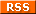feed! Your name Your email (optional) Validation Are you real? Please type 15086 backwards. Your comment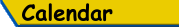Mon Tue Wed Thu Fri Sat Sun «   December 2021   » 1 2 3 4 5 6 7 8 9 10 11 12 13 14 15 16 17 18 19 20 21 22 23 24 25 26 27 28 29 30 31

(Felicity? Marte? Find out!)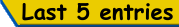List all b.log entries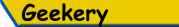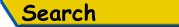Search Rick's b.log!
PS: Don't try to be clever.
It's a simple substring match.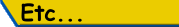Last read at 17:59 on 2023/09/21.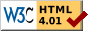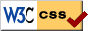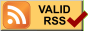© 2021 Rick Murray This web page is licenced for your personal, private, non-commercial use only. No automated processing by advertising systems is permitted. RIPA notice: No consent is given for interception of page transmission.

 Have you noticed the watermarks on pictures? Read the explanation.
Next entry - 2021/12/13